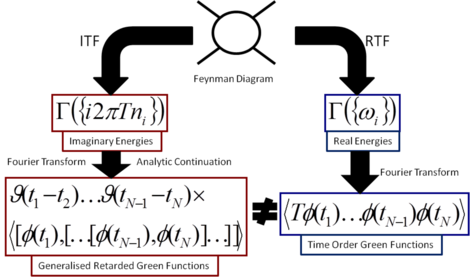# Tim Evans QFT Pages

## Tim Evans' Quantum Field Theory Course 2015 (PT4.4)

The main web site for my QFT course is on the Theory Group web pages, see the QFT Lecture Course web page. However because of CMS bugs hosting the main web site I maintain a copy of parts of this on my QFT Course outline.

## Thermal Field Theory

Thermal Field Theory (TFT), also known as Finite Temperature Field Theory, looks at many body problems where the underlying dynamics of the particles (fundamental or otherwise) is described by quantum field theory. TFT can be applied to cosmology, heavy-ion collisions at CERN, and to condensed matter problems such as Bose-Einstein condensation in cold atoms, superconductivity, and superfluidity. I have worked on both the formal aspects of the theory and on applications in fields such as particle physics and cosmology.

### What is being calculated in Thermal Field Theory?

One part of my interest in the formal aspects is to understand how the different approaches to Thermal Field Theory are related, and how they might be optimal for different problems. In particular in N-Point Finite Temperature Expectation Values at Real Times (Nucl.Phys.B, 374 (1992) 340-370) I showed how to relate the results obtained for real-time Green functions (essential for dynamical processes) found using the two alternative approaches, the Matsubara formalism using imaginary time, and the real-time methods.

There are two distinct approaches to calculations in Thermal Field Theory. One is based on the use of real physical times, called RTF (Real Time Formalism). The other uses unphysical imaginary times, the Matsubara formalism or ITF (Imaginary Time Formalism). ITF is the older, apparently simpler (its not underneath the surface) and more popular approach. The question is how do you reach physical quantities describing dynamics, such as the propagation or interaction of physical particles? Technically, how do you calculate Green functions at real (physical) times?

As RTF is constructed using real times it produces the desired results directly. With the ITF method, one has to perform an analytic continuation of results of its calculations as everything in ITF is expressed in imaginary time. What I showed in N-Point Finite Temperature Expectation Values at Real Times was that while the terms of the calculations in RTF and ITF match, that is the same Feynman diagrams are involved, the results are very different. This had been shown in one simple explicit example before but the reasons for the difference had not been understood. In this paper I not only showed that this mismatch was not a mistake but that it was a general feature. In particular I showed that the analytic continuation of ITF to real times produced different types of real-time Green function (retarded and generalised retarded Green functions) from those obtained in RTF (time-ordered Green functions). Thus the two methods calculate the same dynamical physics but they wrap up the physics in two different forms. I also provided a way to relate the results from the two calculational formalisms. Surprisingly, this relationship was well known for simple propagation (two-point Green functions) but had not been understood in more general cases. My work applied to practical calculations and to the general case.

As part of this work I defined a new sequence of integers, 2, 6, 32, 370, 11292, 1066044, 347326352, 419172756930, etc., which is Sloane sequence A034997 (see also What is being calculated with Thermal Field Theory?). This sequence has since been revisited using different techniques.

The case for three-point functions is the simple yet illustrates many of the main points. This was developed in an earlier paper 3-Point Functions at Finite Temperature in Phys. Lett. B 249 (1990) 286-290. The work also shows how to construct spectral representations of higher-order Green functions in thermal field theory. Again the three-point case is the simplest and was developed in another paper Spectral Representation of Three Point Functions at Finite Temperature Phys. Lett. B 252 (1990) 108-112.(Click on image for larger version)

### Wick's Theorem in Thermal Field Theory

Another aspect of my work on formal aspects has been to look at Wick's theorem in thermal field theory. This is central to the canonical approach to any Quantum Field Theory (usually it is the first method taught) yet to use it in Thermal Field Theory requires a fundamental change in the definition of normal ordering, see Wick's Theorem at Finite Temperature Nucl.Phys. B 474 (1996) 481-496 [hep-ph/9601268].

## Non-perturbative Methods

Thermal field theory requires non-perturbative methods for most calculations, even when the underlying microscopic theory is weakly coupled which normally give reliable results when using simple perturbation theory. Thus am also interested in non-perturbative methods such as large N, lattice Monte Carlo methods and in particular, variational methods such as the linear delta-expansion.

## Multiplicative Anomalies and Zeta Function Renormalisation

I have also studied multiplicative anomalies, strange additional terms found when working in quantum field theory with zeta-function regularisation. In Regularization schemes and the multiplicative anomaly [Phys.Lett.B 457 (1999) 127-132, hep-th/0104128] I showed that there are serious drawbacks in the use of zeta-function regularisation, a popular method used in difficult problems quantum field theory such as the Casimir effect and when working with quantum field theory in strange space-times. In general this does not satisfy the criteria laid down by Wilson for a good regularisation method. Also see Vacuum Energy Densities and Multiplicative Anomalies in a Free Bose Gas arXiv:hep-th/0104128.